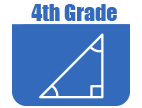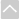Review Activities and Games

These resources are intended for review and practice after initial instruction of the standards.

Strand: Mathematical PRACTICES (4.MP)Strand: OPERATIONS AND ALGEBRAIC THINKING (4.OA)
Use the four operations with whole numbers (addition, subtraction, multiplication, and division) to solve problems (Standards 4.OA.1–3).
Gain familiarity with factors and multiples (Standard 4.OA.4).
• Factor Trail Game
In this lesson students will practice identifying the integer factors of numbers up to 100.
• Factors
This lesson is designed to help students understand factors of whole numbers.
• Finding Factors
This lesson plan's activities give students practice in finding the factors of whole numbers.
• Sets and the Venn Diagram
This lesson is designed to help students understand the ideas surrounding sets and Venn diagrams.
Generate and analyze numeric and shape patterns (Standard 4.OA.5).Strand: NUMBER AND OPERATIONS IN BASE TEN (4.NBT)
Generalize place value understanding for multi-digit whole numbers by analyzing patterns, writing whole numbers in a variety of ways, making comparisons, and rounding (Standards 4.NBT.1–3).
Use place value understanding and properties of operations to perform multidigit addition, subtraction, multiplication, and division using a one-digit divisor (Standards 4.NBT.4–6) Expectations in this strand are limited to whole numbers less than or equal to 1,000,000.
• Arithmetic Four
In this game for two players, students solve problems involving whole number and integer addition, subtraction, multiplication, and division.
• Order of Operations Four
This applet pits two players against each other in solving expressions using the four basic operations.Strand: NUMBER AND OPERATIONS - FRACTIONS (4.NF)
Extend understanding of equivalence and ordering of fractions (Standards 4.NF.1–2)
• Comparing Fractions with Lines
This lesson will help students simplify fractions, compare and order them on a number line, and estimate their value.
• Equivalent Fractions Finder
Students doing this lesson will visually experiment with the relationship between the value of fractions and areas within a square or a circle. In doing so they will practice simplifying fractions and compare fractions.
Build fractions from unit fractions by applying and extending previous understandings of operations on whole numbers (Standards 4.NF.3–4)
• Comparing Fractions with Lines
This lesson will help students simplify fractions, compare and order them on a number line, and estimate their value.
• Comparing Two Different Pizzas
Students are given the example of two whole pizzas, one large and one medium. They must determine what fraction of the two pizzas has been eaten if someone eats 2 slices of the medium pizza. They must explain their reasoning and draw a picture to illustrate their solution.
• Histograms and Bar Graphs
This lesson will introduce students to histograms and bar graphs and help them understand the difference between them.
• Making 22 Seventeenths in Different Ways
This is a straightforward task related to adding fractions with the same denominator. The main purpose is to emphasize that there are many ways to decompose a fraction as a sum of fractions, similar to decompositions of whole numbers that students should have seen in earlier grades. It is suitable for assessment or, with opportunity for classroom discussion, it could also be useful in instruction.
• Multiply Fractions Jeopardy
This game can be played by one or two players as they solve multiplication problems with fractions.
• Peaches
This task provides a context where it is appropriate for students to subtract fractions with a common denominator; it could be used for either assessment or instructional purposes.
• Plastic Building Blocks
The purpose of this task is to have students add mixed numbers with like denominators when given a problem in the context of two students building a castle out of building blocks.
• Single Fraction Finder
In this lesson students will practice dividing a whole into a fraction and visualize fractions on a number line.
• Subtraction of Fractions Using a Visual Model
Students will learn how to subtract fractions with unlike denominators by using a visual model. NOTE: You have to create a Free PBS Account to view this web page, but it is easy to do and worth the effort.
• What Fraction of this Shape is Red?
This Teaching Channel video shows how students can explore part and whole by creating pattern block designs. (4 minutes)
• Writing a Mixed Number as an Equivalent Fraction
This task relates to writing a mixed number as an equivalent fraction. The purpose of this task is to help students understand and articulate the reasons for the steps in the usual algorithm for converting a mixed number into an equivalent fraction,
Understand decimal notation to the hundredths and compare decimal fractions with denominators of 10 and 100 (Standards 4.NF.5– 7). Denominators for fourth grade are limited to 2, 3, 4, 5, 6, 8, 10, 12, and 100.Strand: MEASUREMENT AND DATA (4.MD)
Solve problems involving measurement and conversion of measurements from a larger unit to a smaller unit (Standards 4.MD.1–2)
• IXL Game: Measurement: Convert mixed customary units
This game helps fourth graders understand relative sizes of measurement units within one system of units. This is just one of many online games that supports the Utah Math core. Note: The IXL site requires subscription for unlimited use.
• Smile Metric Style
In this activity students work with the metric system by measuring each other's smiles.
• The Iditarod & Math
This Teaching Channel video and lesson plan will help students calculate elapsed time and distance using real-time data. (7 minutes)
• What Does it Mean to Measure?
This lesson plan asks students to measure properties of various objects including weight, area, and volume and determine the appropriate metric unit for taking the measurements.
Apply knowledge of area and perimeter to solve realworld and mathematical problems (Standard 4.MD.3)
• Area (elementary)
In this lesson students will find the area of rectangular shapes and irregular shapes with right angles.
Represent and interpret data through the use of a line plot (Standard 4.MD.4)
Understand various concepts of angles and angle measurement (Standard 4.MD.5–7)
• Angles (elementary)
This lesson is designed to help students learn about classifying angles by their measure.Strand: GEOMETRY (4.G.)
Draw and identify lines and angles, as well as classify shapes by properties of their lines and angles (Standards 4.G.1–3)
In this lesson the student is introduced to parallelograms, rectangles, and trapezoids and practices creating various types of quadrilaterals.
• Angles (elementary)
This lesson is designed to help students learn about classifying angles by their measure.
• Area Explorations
In this lesson, students will explore the area of irregular shapes to find multiple different methods for calculating area
• Floor Tiles
This lesson will help students identify and examine symmetry in geometric figures as well as describe and classify polygons.
• IXL Game: Geometry: Parallel, perpendicular, intersecting
This game helps fourth graders draw points, lines, line segments, rays, angles, and perpendicular and parallel lines. This is just one of many online games that supports the Utah Math core. Note: The IXL site requires subscription for unlimited use.
• Sets and The Venn Diagram (Beginner)
By introducing students to the idea of a set, this lesson will help students learn about the vocabulary of sets and Venn diagrams, as well as how to arrange things in a Venn diagram.
• Tessellate!
This lesson and activity will help the student understand how to classify two-dimensional figures based on properties. The student manipulates shapes to create now polygons.The Online Core Resource pages are a collaborative project between the Utah State Board of Education and the Utah Education Network. If you would like to recommend a high quality resource, contact Trish French (Elementary) or Lindsey Henderson (Secondary). If you find inaccuracies or broken links contact resources@uen.org.